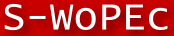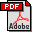Scandinavian Working Papers in Economics

# No 2003:17: The Impact of Estimation Error on Portfolio Selection for Investors with Constant Relative Risk Aversion

Christoffer Bengtsson
Christoffer Bengtsson: Department of Economics, Lund University, Postal: Department of Economics, School of Economics and Management, Lund University, Box 7082, S-220 07 Lund, Sweden

Abstract: This paper examines the impact of estimation error in a simple single-period portfolio choice problem when the investor has power utility and asset returns are jointly lognormally distributed. These assumptions imply that such an investor selects portfolios using a modified mean-variance framework where the parameters that he has to estimate are the mean vector of log returns and the covariance matrix of log returns. Following Chopra and Ziemba (1993), I simulate estimation error in what are assumed to be the true mean vector and the true covariance matrix and the impact of estimation error is measured in terms of percentage cash equivalence loss for the investor. To obtain estimation error sizes that are similar to the estimation error sizes in actual estimates, I use a Bayesian approach and Markov Chain Monte Carlo Methods. The empirical results differ significantly from Chopra and Ziemba (1993), suggesting that the effect of estimation error may have been overestimated in the past. Furthermore, the results tend to question the traditional viewpoint that estimating the covariance matrix correctly is strictly less important than estimating the mean vector correctly.

JEL-codes: G11

26 pages, First version: November 10, 2003. Revised: April 29, 2004.

Full text files

`WP03_17.pdf`

Questions (including download problems) about the papers in this series should be directed to Prakriti Thami ()
Report other problems with accessing this service to Sune Karlsson ().